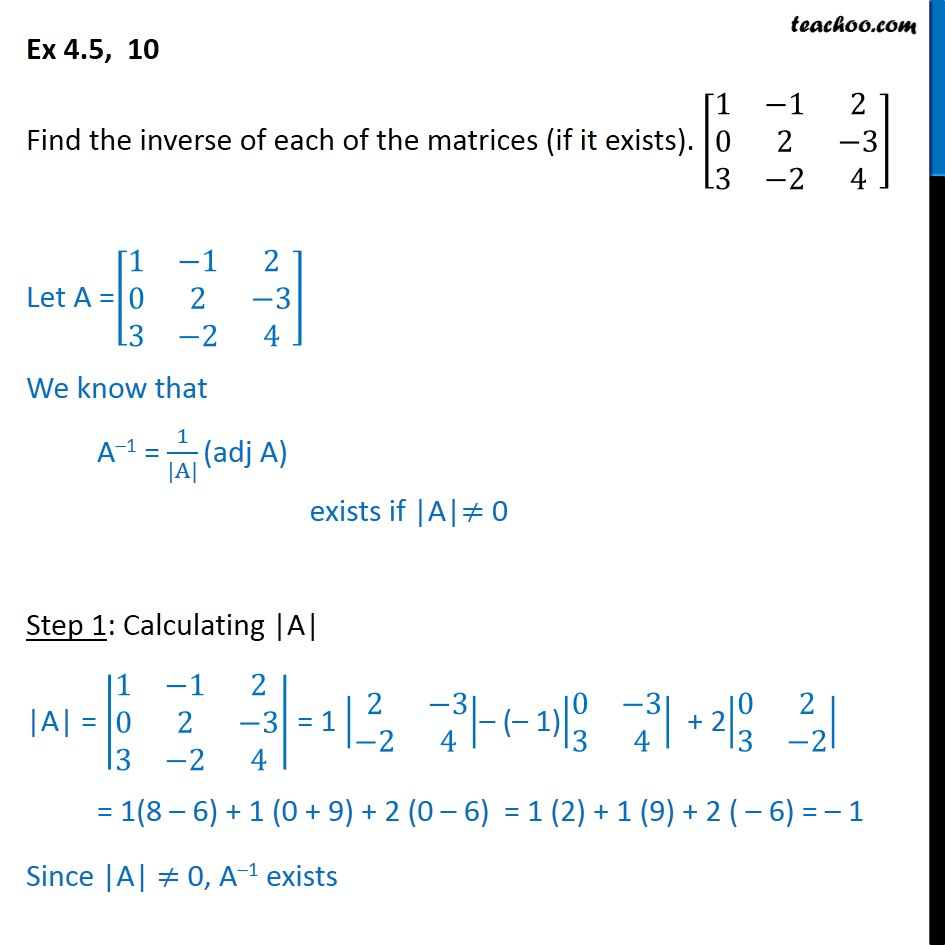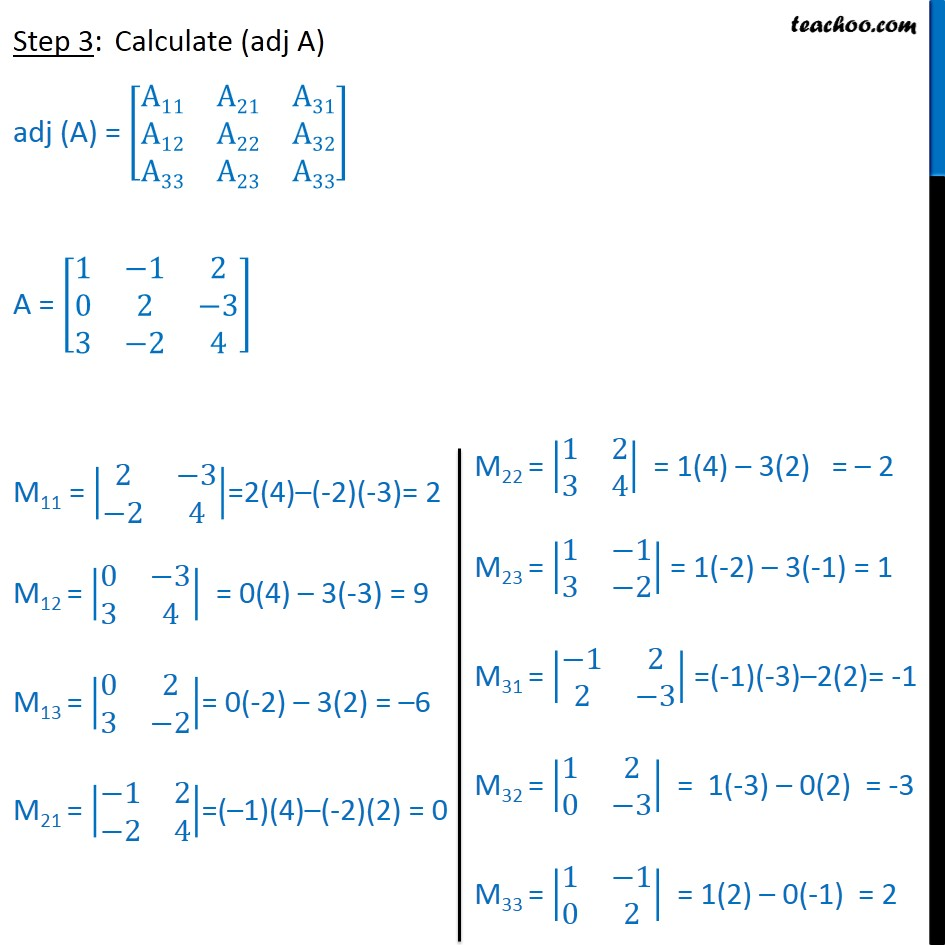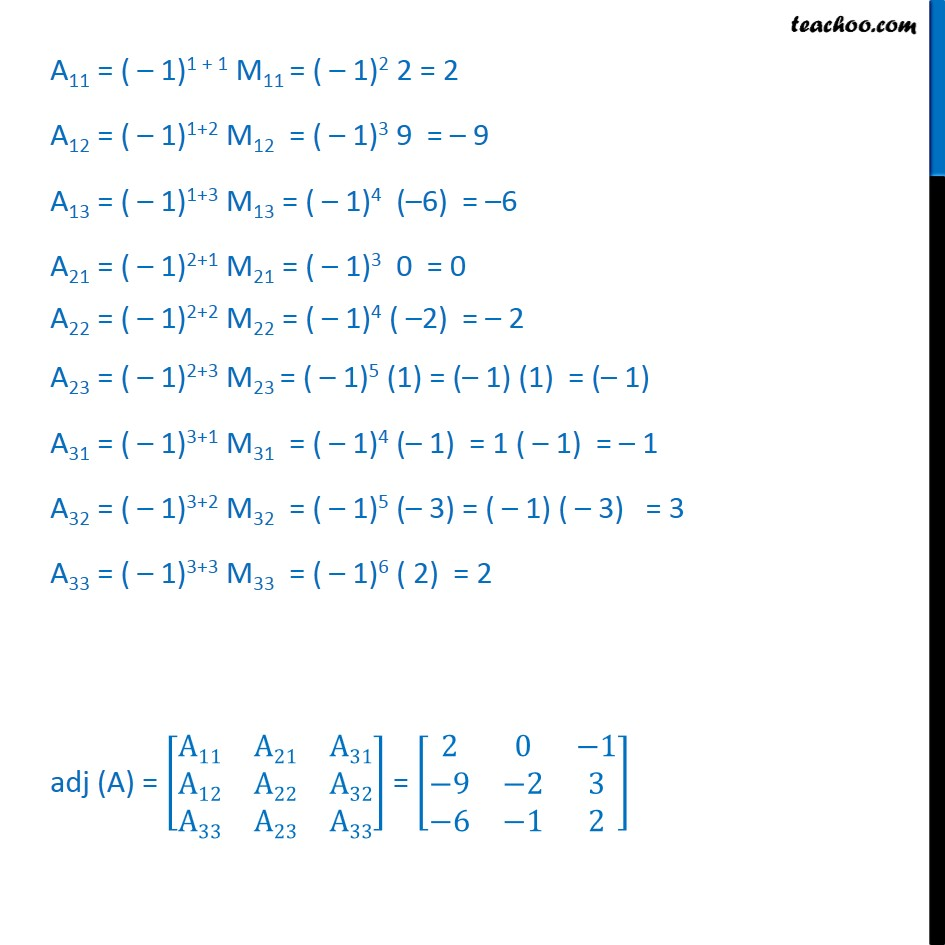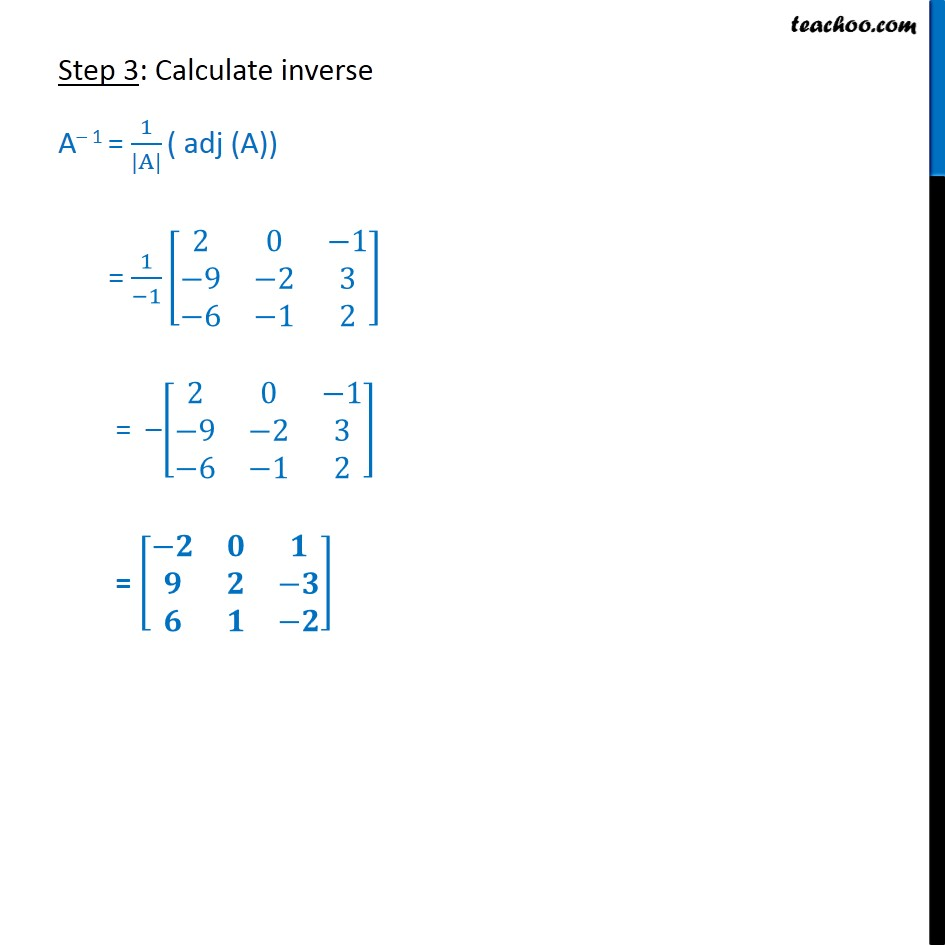1. Class 12
2. Important Question for exams Class 12
3. Chapter 4 Class 12 Determinants

Transcript

Ex 4.5, 10 Find the inverse of each of the matrices (if it exists). 1 1 2 0 2 3 3 2 4 Let A = 1 1 2 0 2 3 3 2 4 We know that A 1 = 1 |A| (adj A) exists if |A| 0 Step 1: Calculating |A| |A| = 1 1 2 0 2 3 3 2 4 = 1 2 3 2 4 ( 1) 0 3 3 4 + 2 0 2 3 2 = 1(8 6) + 1 (0 + 9) + 2 (0 6) = 1 (2) + 1 (9) + 2 ( 6) = 1 Since |A| 0, A 1 exists Step 3: Calculate (adj A) adj (A) = A11 A21 A31 A12 A22 A32 A33 A23 A33 A = 1 1 2 0 2 3 3 2 4 M11 = 2 3 2 4 =2(4) (-2)(-3)= 2 M12 = 0 3 3 4 = 0(4) 3(-3) = 9 M13 = 0 2 3 2 = 0(-2) 3(2) = 6 M21 = 1 2 2 4 =( 1)(4) (-2)(2) = 0 A11 = ( 1)1 + 1 M11 = ( 1)2 2 = 2 A12 = ( 1)1+2 M12 = ( 1)3 9 = 9 A13 = ( 1)1+3 M13 = ( 1)4 ( 6) = 6 A21 = ( 1)2+1 M21 = ( 1)3 0 = 0 A22 = ( 1)2+2 M22 = ( 1)4 ( 2) = 2 A23 = ( 1)2+3 M23 = ( 1)5 (1) = ( 1) (1) = ( 1) A31 = ( 1)3+1 M31 = ( 1)4 ( 1) = 1 ( 1) = 1 A32 = ( 1)3+2 M32 = ( 1)5 ( 3) = ( 1) ( 3) = 3 A33 = ( 1)3+3 M33 = ( 1)6 ( 2) = 2 adj (A) = A11 A21 A31 A12 A22 A32 A33 A23 A33 = 2 0 1 9 2 3 6 1 2 Step 3: Calculate inverse A 1 = 1 |A| ( adj (A)) = 1 1 2 0 1 9 2 3 6 1 2 = 2 0 1 9 2 3 6 1 2 =

Chapter 4 Class 12 Determinants

Class 12
Important Question for exams Class 12

About the AuthorDavneet Singh
Davneet Singh is a graduate from Indian Institute of Technology, Kanpur. He has been teaching from the past 9 years. He provides courses for Maths and Science at Teachoo.